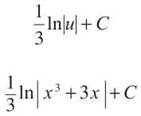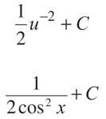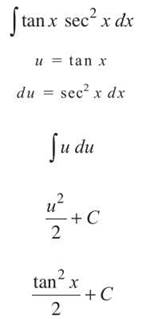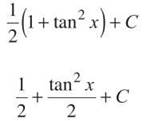﻿ ﻿U-SUBSTITUTION - Integration - AP CALCULUS AB & BC REVIEW - Master AP Calculus AB & BC

## Master AP Calculus AB & BC

Part II. AP CALCULUS AB & BC REVIEW

CHAPTER 7. Integration

U-SUBSTITUTION

NOTE. Remember, there is no u-substitution without you. Isn’t that nice? Do you feel all fuzzy inside? No? Me neither, so get used to it.

Until this point, you have been able to integrate painfully few things. For example, you can solve ∫ cos x dx, but not ∫ cos 5x dx. If you thought ∫ cos 5x dx = ∫ sin 5x dx, all you have to do is take the derivative (to check):Since the result was not cos 5x, our antiderivative of sin 5x doesn’t check out. How- ever, we were pretty close.

In order to integrate things like cos 5x or e2x, you need to employ a method called u-substitution. This method allows you to integrate composite functions, sort of like the Chain Rule allowed you to differentiate composite functions. Here’s a good rule of thumb: If you would use the Chain Rule to take the derivative of an expression, you should use u-substitution to integrate it. How important is «-substitution? It is all over the AP test, and it is an essential skill you’ll use for the remainder of the year. Let’s begin by integrating the above examples using w-substitution.

Example 11: Integrate the following:

(a) ∫ cos 5x dx

If this were cos x, you could integrate it. Therefore, we will introduce a new variable, u, like so: u = 5x. This way, the expression will become cos u, and we know the integral of cos u—it is sin u. However, before we get ahead of ourselves, we need to find the derivative of u (with respect to x); this is the all-important second step.

du =5 dx

We want to solve this for dx. Why? We will be replacing x’s with u’s in the integral, so we want to replace dx's with du's so all the variables match. Solving for dx is very easy:Now, substitute u = 5x andinto the original integral to getAt this point, you can pull the 1/5 out of the integral since it’s just a constant and the result is beautiful:The integral of cos u is sin u, so you getHowever, your final answer can’t include ids since the original problem didn’t include u’s. To finish, substitute in the original value for u, u = 5x.(b)We can integrate ex, but not e2x. Because the 2x is not a single variable (which we want), we replace it with a single variable, u:

u = 2x

du = 2dx

Again, solve for dx so we can replace it in the integral and make the variables match.Now, substitute into the integral, make those x’s a bad memory, and factor out that 1/2.Nothing is easier to integrate than eu!Get everything back to x’s and you’re finished.If you don’t feel confident that these answers are correct, take their derivatives and check them.

So far, you’ve seen that «-substitution can take the place of the “inner function” of a composite function and make the integral simpler by replacing that troublesome inner function with a single variable. But, this is only one good use of u-substitution. Often, you’ll see a complex integral problem with this specific characteristic: part of the integral’s derivative is also in the problem. For example, consider this integral:Of the two pieces in the integral, whose derivative is present? The answer is ln x. We can rewrite the integral to look like this:The derivative of ln x is 1/x, and it’s in the integral! Why is this important? Watch and see.

Example 12: EvaluateSolution: Because, as we said above, In x has its derivative in the integral, we set it equal to u (and take the derivative as we did each time in Example 11).

u = ln xThat’s why it’s important that the derivative was also present. Because we could rewrite the original integral expression as(as we showed above), we can now replacewith du. Why? Because we found out they were equal when we differentiated the statement u =lIn x. By substitution, our original integral becomesNOTE. If you can’t integrate by simple means (like the Power Rule for Integration), immediately try u-substitution. Make it your on-deck batter.

This is really easy to integrate:Simply replace the ids with x’s, and you’re finished. The final answer isThe hardest part of w-substitution is deciding what the u should be. If you try a few things and they don’t work, don’t get discouraged. Try other things. Eventually, you’ll find something that works.

5 Steps to Success with U-Substitution

1. Choose part of the integral expression to be your u. This is the diabolical part. If you’re dealing with a composite function, you might want to set u equal to the inner function. If an expression f’(x) and its derivative f(x) are both in a function, set u equal to f(x). There are no hard and fast rules. Practice is the key.

2. Find the derivative of the u expression with respect to x (or whatever the variable present is).

3. If necessary, solve the derivative you found in step 2 for dx.

4. Substitute back into the original integral and integrate.

5. Replace your it's using your original u statement from number 1.

Example 13: Solve the following integrals using u-substitution.

(a)This looks complicated. You might try a couple of things for u, but if you set u = x3 + 3x, watch what happens:TIP. If you cannot figure out what u should be and the integral expression is a fraction, try to set u equal to the denominator, as in Example 13(a).

The entire numerator and the dx get replaced with du/3, and the original integral expression becomesand you can integrate this quite easily.b)There are at least two good ways to solve this, both using u-substitution.

Method One: Rewrite tan x asMethod Two: Rewriteas sec2 x.Although those answers do not immediately look the same, they are equivalent. If you need proof, here it is:Use Pappa to substitute 1 + tan2 x for sec2 x.Realize that ½ + C is simply another constant, or a different C, and the expressions are equal. There is never a guarantee that two C’s are equal, even though we use the same variable to represent them—C is an arbitrary constant.You can also use u-substitution in definite integrals. The only adjustment you must make is that your limits of integration (which are x limits) must become u limits. It’s very easy to accomplish this.

Example 14: EvaluateSolution: Begin by pulling the constant 4 out of the integral to getNow, set it equal to the value inside the radical.ALERT! When you change the x boundaries to u boundaries, the lower bound might end up higher than the upper bound. If this happens, don’t panic and don’t change them! Leave them as is, and complete the problem.

At this point, it is incorrect to writeThe boundaries 2 and 3 are x boundaries. We have to make all the variables match (which is why dx has to become du.) Therefore, plug each of these x values into the u expression u = 3x3 - 5 to get the corresponding u boundary:Therefore, we can rewrite the original integral asIntegrate and apply the Fundamental Theorem to finish.The last important topic of this section (and it was a long one, wasn’t it?) is integrating trigonometric functions. You already know the integrals of sin x and cos x, but you don’t know the other four, and it’s important that you know all six. It is actually quite easy to find ∫ tan x dx. If you rewrite it in terms of sine and cosine, you can integrate using u-substitution:TIP. It sometimes is useful to set u equal to the part of your integral that is raised to a power. In Example 14, 3x3 - 5 is raised to the 1/2 power.

You can integrate cotangent in a similar way to getIntegrating sec x and esc x are a little more difficult and require a trick or two, so we won’t get into that. However, it is important that you know what their integrals are, if not where they came from:Just like you memorized the derivatives of these functions, it’s equally important to memorize their integrals. Except for sine and cosine, all of the trig integrals contain “ln.” To help memorize ∫ sec x dx, remember its derivative,and ∫ sec x dx = ln |sec x + tan x| + C.

One multiplies the terms, and the other adds them. The same goes for csc x. You really shouldn’t need a trick to memorize the integrals for tan x and cot x; as you’ve seen with tan x, they are very easy to find with u-substitution.

EXERCISE 7

Directions: Solve each of the following problems. Decide which is the best of the choices given and indicate your responses in the book.

DO NOT USE A GRAPHING CALCULATOR FOR ANY OF THESE PROBLEMS.

Evaluate the following integrals.1. This is a composite function, so set u = 3x + 14, the inner function. (This also follows the earlier tip that something to a power can be the u.)

u = 3x + 14

Therefore, the derivative is du = 3dx and du/3 = dx. The new u boundaries will be

u = 3(0) + 14 = 14 and

u = 3(3) + 14 = 23

The integral then becomes2. This is a fraction that contains a composite function. The inner function is e-x so make that your u:The —du will replace the denominator as well as dx so you can integrate.3. The best u in this problem is u = cos 5x. Therefore, du = —5 sin 5x dx, and —1/5du = sin 5x dx.4. Another composite function: Set u = x/2, so du = 1/2dx and 2du = dx. The new u-boundaries will beand the new integral will be5. You could set u = sin 3x, but if you set u = esin 3x, watch what happens:All of the integral disappears, to be replaced with du/3.Of course, the integral of du is u, so the answer isThis is a great technique for integrating any kind of exponential function; it works like magic.

6. The 1/ln3 is merely a constant we can pull out of the integral; next, set u = x2 + 4. This results in du = 2x dx, and du/2 = x dx. When you substitute back you getThere’s no need to use absolute values for the natural log, since x2 + 4 will always be positive. You might also apply log properties and write the answer as﻿